### Construction of SFG from simultaneous equations

Construction of SFG from simultaneous equations

Rules For Construction of a Signal Flow Graph:

1. The actual signal only travels through branch and towards the direction specified through an arrow indicator.

2. The Output from a branch is the product of the gain and input of that branch.

3. Input to a specific node is the sum of all the signals that enter the node.

Let us consider the following set of simultaneous equations:
=

=
=

There are total 6 variables and 7 coefficients, i.e there will be 6 nodes and 7 branches.
Signal Flow Graph for equation = :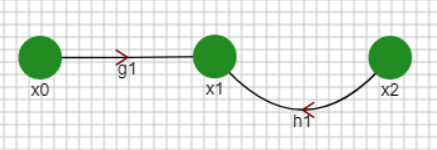Signal Flow Graph for equation is given by: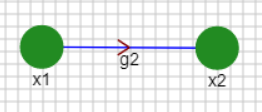Signal Flow Graph for equation = is given by: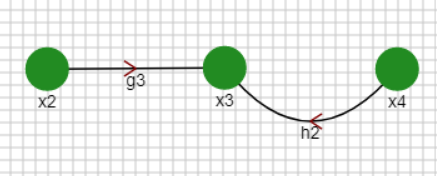Signal Flow Graph for =: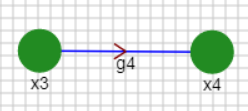Signal Flow Graph for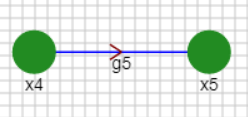Combining All the Signal Flow Graph we get: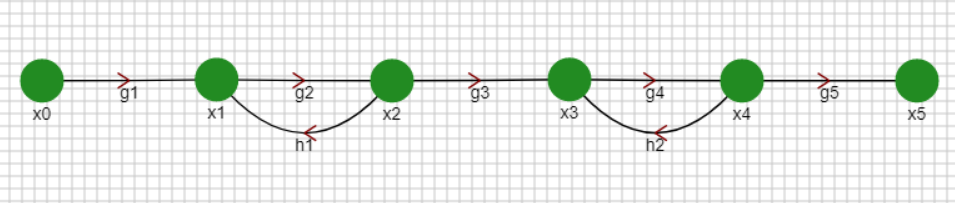Signal Flow Graph From Block Diagram

We can convert a Block Diagram Representation into a Signal Flow Graph Representation using the following steps :

1. Convert all the variables, summing points and takeoff points to nodes.

2. Represent all the blocks as branches and represent the functions in the block as transmittance of the branch.

3. Connection of nodes and branches has to remain the same and if there is any connection without block, represent it  a branch with unity gain.